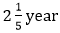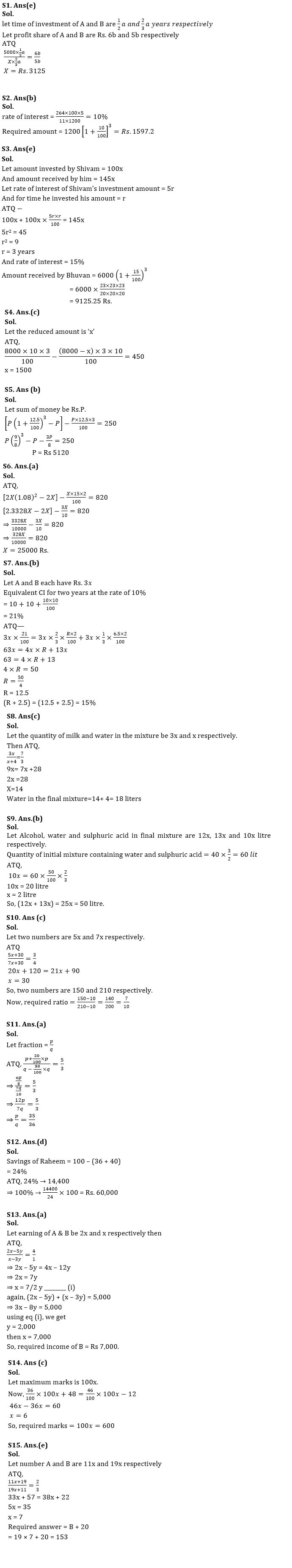Latest Banking jobs   »   Quantitative Aptitude Quiz For SBI, IBPS...

# Quantitative Aptitude Quiz For SBI, IBPS Prelims 2021- 14th March

Q1. A and B invested in a partnership Rs. 5000 and Rs. X for half and two-third of time respectively and profit share of A is 20% more than profit share of B. Find X?
(a)       Rs. 3000
(b)      Rs. 2000
(c)       Rs. 2525
(d)      Rs. 2715
(e)       Rs. 3125

Q2. Simple interest on a sum of Rs. 1200 afteris Rs. 264. Find amount incurred on same rate of interest compounded annually on same sum after 3 years?
(a)       Rs. 1445.5
(b)      Rs. 1597.2
(c)       Rs.1582.2
(d)      Rs. 1682.8
(e)       None of these.

Q3. Shivam invested some amount at SI and gets 45% more amount and the ratio between numerical value of rate of interest of Shivam’s investment to time period of Shivam’s investment is 5 : 1. If Bhuvan invested Rs. 6000 at the same rate and same period of time on CI, then find he will get how much amount?
(a) 9075.25 Rs
(b) 9175.25 Rs
(c) 8925.25 Rs
(d) 8975.25 Rs
(e) 9125.25 Rs

Q4.      Bhavya prepare to lend 8000 Rs for 3 years at rate of 10% p.a. but at the time of lending he lends less amount due to which his total gain decreases by 450 Rs find the amount he reduced from the initial amount(in Rs)
(a) 6500
(b) 2000
(c) 1500
(d) 4000
(e) 2500

Q5. Find the sum if the difference between simple interest and compound interest on that sum of money for 3 years at 12.5% per annum is Rs 250.
(a) Rs 4210
(b) Rs 5120
(c) Rs 4260
(d) Rs 5210
(e) Rs 6230

Q6. A man invested Rs. X and Rs. 2X at 15% simple interest and at 8% compound interest (compounding annually) for two years respectively. Difference between simple interest and compound interest received after two years is Rs.820. Find the value of X.
(a) Rs.25000
(b) Rs.30600
(c) Rs.28600
(d) Rs.22200
(e) Rs.26200

Q7. A and B have equal amount, while A invested his amount on C.I. for two years at the rate of 10% p.a. and B invested 2/3rd of his amount at the rate of R% p.a. on simple interest and remaining amount at the rate of 6.5% p.a. on also simple interest. If interest received by both at the end of two years are equal, then find the value of (R + 2.5) %?
(a) 12%
(b) 15%
(c) 17.5%
(d) 22.5%
(e) 12.5%

Q8. A mixture contains milk and water in the ratio 3:1. On adding 4 liters of water, the ratio of milk to water becomes 7:3. The quantity of water in the final mixture is?
(a) 16 liters
(b) 14 liters
(c) 18 liters
(d) 20 liters
(e) 24 liters

Q9. 50% of a mixture containing water and Sulphuric acid in ratio 1 : 2 is mixed with 40% of another mixture containing alcohol and water in ratio 3 : 2 so that ratio of alcohol: water : sulphuric acid becomes 12 : 13 : 10 in the final mixture. If sulphuric acid in initial mixture is 40 liter, Find total quantity of water and alcohol in final mixture?
(a) 25 liters
(b) 50 liters
(c) 75 liters
(d) 15 liters
(e) None of these

Q10. Ratio of two numbers is 5 : 7. If 30 is added in each number, the ratio becomes 3 : 4. Find the ratio of the number if 10 is subtracted from each number.
(a) 5 : 8
(b) 8 : 11
(c) 7 : 10
(d) 6 : 11
(e) 7 : 9

Q11. A fraction becomes 5/3 when 20% of numerator is added to its numerator and 30% of denominator is subtracted from its denominator. Find the fraction.
(a)  35/36
(b)  36/25
(c)  33/35
(d)  27/35
(e)  35/33

Q12. Raheem spends 36% of his monthly income on daily spendings, 40% on house rent and children fee together and rest amount he saves for future needs. If his total monthly savings be Rs. 14,400, then find his total monthly income.
(a) Rs. 45,000
(b) Rs. 40,000
(c) Rs. 48,000
(d) Rs. 60,000
(e) Rs. 55,000

Q13. A and B earn in the ratio 2 : 1. They spend in the ratio 5 : 3 and save in the ratio 4 : 1. If the total monthly savings of both A and B are Rs. 5,000, the monthly income of B is
(a) Rs. 7,000
(b) Rs. 14,000
(c) Rs. 5,000
(d) Rs. 10,000
(e)12,000

Q14. In an examination, Dharam got 36% of maximum marks and failed by 48 marks, while Ankit got 46% of maximum marks which is 12 more than passing marks. Find the maximum marks.
(a) 500
(b) 750
(c) 600
(d) 450
(e) 650

Q15. Two numbers A and B are in ratio 11 : 19, when 19 is added to A and 11 is added to B the ratio becomes 2 : 3, Find value of (20 + B)?
(a) 154
(b) 144
(c) 164
(d) 133
(e) 153

Practice More Questions of Quantitative Aptitude for Competitive Exams:

###### Study Plan for IBPS and SBI Exams 2021

SolutionsPractice with Online Test Series for SBI and IBPS Prelims 2021:×

Thank You, Your details have been submitted we will get back to you.Join India's largest learning destination

What You Will get ?

•Job Alerts
•Daily Quizzes
•Subject-Wise Quizzes
•Current Affairs
•Previous year question papers
•Doubt Solving session

ORJoin India's largest learning destination

What You Will get ?

•Job Alerts
•Daily Quizzes
•Subject-Wise Quizzes
•Current Affairs
•Previous year question papers
•Doubt Solving session

ORJoin India's largest learning destination

What You Will get ?

•Job Alerts
•Daily Quizzes
•Subject-Wise Quizzes
•Current Affairs
•Previous year question papers
•Doubt Solving session

Enter the email address associated with your account, and we'll email you an OTP to verify it's you.Join India's largest learning destination

What You Will get ?

•Job Alerts
•Daily Quizzes
•Subject-Wise Quizzes
•Current Affairs
•Previous year question papers
•Doubt Solving session

Enter OTP

Please enter the OTP sent to
/6

Did not recive OTP?

Resend in 60sJoin India's largest learning destination

What You Will get ?

•Job Alerts
•Daily Quizzes
•Subject-Wise Quizzes
•Current Affairs
•Previous year question papers
•Doubt Solving sessionJoin India's largest learning destination

What You Will get ?

•Job Alerts
•Daily Quizzes
•Subject-Wise Quizzes
•Current Affairs
•Previous year question papers
•Doubt Solving session

Almost there

+91Join India's largest learning destination

What You Will get ?

•Job Alerts
•Daily Quizzes
•Subject-Wise Quizzes
•Current Affairs
•Previous year question papers
•Doubt Solving session

Enter OTP

Please enter the OTP sent to Edit Number

Did not recive OTP?

Resend 60

By skipping this step you will not recieve any free content avalaible on adda247, also you will miss onto notification and job alerts

Are you sure you want to skip this step?

By skipping this step you will not recieve any free content avalaible on adda247, also you will miss onto notification and job alerts

Are you sure you want to skip this step?